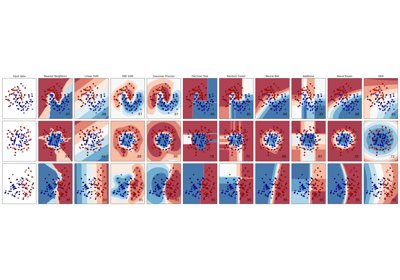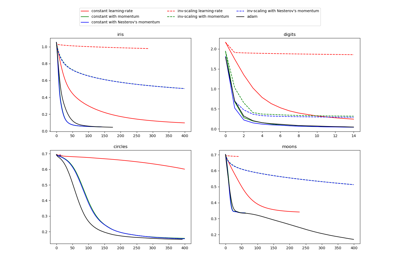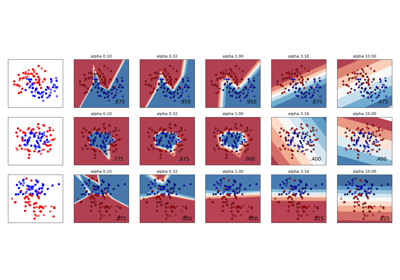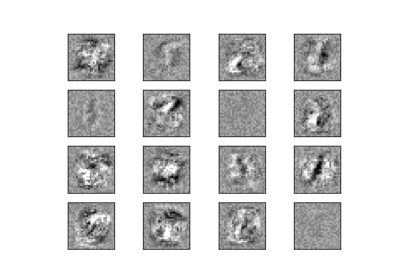# sklearn.neural_network.MLPClassifier¶

class sklearn.neural_network.MLPClassifier(hidden_layer_sizes=(100,), activation='relu', *, solver='adam', alpha=0.0001, batch_size='auto', learning_rate='constant', learning_rate_init=0.001, power_t=0.5, max_iter=200, shuffle=True, random_state=None, tol=0.0001, verbose=False, warm_start=False, momentum=0.9, nesterovs_momentum=True, early_stopping=False, validation_fraction=0.1, beta_1=0.9, beta_2=0.999, epsilon=1e-08, n_iter_no_change=10, max_fun=15000)[source]

Multi-layer Perceptron classifier.

This model optimizes the log-loss function using LBFGS or stochastic gradient descent.

New in version 0.18.

Parameters:
hidden_layer_sizesarray-like of shape(n_layers - 2,), default=(100,)

The ith element represents the number of neurons in the ith hidden layer.

activation{‘identity’, ‘logistic’, ‘tanh’, ‘relu’}, default=’relu’

Activation function for the hidden layer.

• ‘identity’, no-op activation, useful to implement linear bottleneck, returns f(x) = x

• ‘logistic’, the logistic sigmoid function, returns f(x) = 1 / (1 + exp(-x)).

• ‘tanh’, the hyperbolic tan function, returns f(x) = tanh(x).

• ‘relu’, the rectified linear unit function, returns f(x) = max(0, x)

The solver for weight optimization.

• ‘lbfgs’ is an optimizer in the family of quasi-Newton methods.

• ‘sgd’ refers to stochastic gradient descent.

• ‘adam’ refers to a stochastic gradient-based optimizer proposed by Kingma, Diederik, and Jimmy Ba

Note: The default solver ‘adam’ works pretty well on relatively large datasets (with thousands of training samples or more) in terms of both training time and validation score. For small datasets, however, ‘lbfgs’ can converge faster and perform better.

alphafloat, default=0.0001

Strength of the L2 regularization term. The L2 regularization term is divided by the sample size when added to the loss.

batch_sizeint, default=’auto’

Size of minibatches for stochastic optimizers. If the solver is ‘lbfgs’, the classifier will not use minibatch. When set to “auto”, batch_size=min(200, n_samples).

Learning rate schedule for weight updates.

• ‘constant’ is a constant learning rate given by ‘learning_rate_init’.

• ‘invscaling’ gradually decreases the learning rate at each time step ‘t’ using an inverse scaling exponent of ‘power_t’. effective_learning_rate = learning_rate_init / pow(t, power_t)

• ‘adaptive’ keeps the learning rate constant to ‘learning_rate_init’ as long as training loss keeps decreasing. Each time two consecutive epochs fail to decrease training loss by at least tol, or fail to increase validation score by at least tol if ‘early_stopping’ is on, the current learning rate is divided by 5.

Only used when solver='sgd'.

learning_rate_initfloat, default=0.001

The initial learning rate used. It controls the step-size in updating the weights. Only used when solver=’sgd’ or ‘adam’.

power_tfloat, default=0.5

The exponent for inverse scaling learning rate. It is used in updating effective learning rate when the learning_rate is set to ‘invscaling’. Only used when solver=’sgd’.

max_iterint, default=200

Maximum number of iterations. The solver iterates until convergence (determined by ‘tol’) or this number of iterations. For stochastic solvers (‘sgd’, ‘adam’), note that this determines the number of epochs (how many times each data point will be used), not the number of gradient steps.

shufflebool, default=True

Whether to shuffle samples in each iteration. Only used when solver=’sgd’ or ‘adam’.

random_stateint, RandomState instance, default=None

Determines random number generation for weights and bias initialization, train-test split if early stopping is used, and batch sampling when solver=’sgd’ or ‘adam’. Pass an int for reproducible results across multiple function calls. See Glossary.

tolfloat, default=1e-4

Tolerance for the optimization. When the loss or score is not improving by at least tol for n_iter_no_change consecutive iterations, unless learning_rate is set to ‘adaptive’, convergence is considered to be reached and training stops.

verbosebool, default=False

Whether to print progress messages to stdout.

warm_startbool, default=False

When set to True, reuse the solution of the previous call to fit as initialization, otherwise, just erase the previous solution. See the Glossary.

momentumfloat, default=0.9

Momentum for gradient descent update. Should be between 0 and 1. Only used when solver=’sgd’.

nesterovs_momentumbool, default=True

Whether to use Nesterov’s momentum. Only used when solver=’sgd’ and momentum > 0.

early_stoppingbool, default=False

Whether to use early stopping to terminate training when validation score is not improving. If set to true, it will automatically set aside 10% of training data as validation and terminate training when validation score is not improving by at least tol for n_iter_no_change consecutive epochs. The split is stratified, except in a multilabel setting. If early stopping is False, then the training stops when the training loss does not improve by more than tol for n_iter_no_change consecutive passes over the training set. Only effective when solver=’sgd’ or ‘adam’.

validation_fractionfloat, default=0.1

The proportion of training data to set aside as validation set for early stopping. Must be between 0 and 1. Only used if early_stopping is True.

beta_1float, default=0.9

Exponential decay rate for estimates of first moment vector in adam, should be in [0, 1). Only used when solver=’adam’.

beta_2float, default=0.999

Exponential decay rate for estimates of second moment vector in adam, should be in [0, 1). Only used when solver=’adam’.

epsilonfloat, default=1e-8

n_iter_no_changeint, default=10

Maximum number of epochs to not meet tol improvement. Only effective when solver=’sgd’ or ‘adam’.

New in version 0.20.

max_funint, default=15000

Only used when solver=’lbfgs’. Maximum number of loss function calls. The solver iterates until convergence (determined by ‘tol’), number of iterations reaches max_iter, or this number of loss function calls. Note that number of loss function calls will be greater than or equal to the number of iterations for the MLPClassifier.

New in version 0.22.

Attributes:
classes_ndarray or list of ndarray of shape (n_classes,)

Class labels for each output.

loss_float

The current loss computed with the loss function.

best_loss_float or None

The minimum loss reached by the solver throughout fitting. If early_stopping=True, this attribute is set to None. Refer to the best_validation_score_ fitted attribute instead.

loss_curve_list of shape (n_iter_,)

The ith element in the list represents the loss at the ith iteration.

validation_scores_list of shape (n_iter_,) or None

The score at each iteration on a held-out validation set. The score reported is the accuracy score. Only available if early_stopping=True, otherwise the attribute is set to None.

best_validation_score_float or None

The best validation score (i.e. accuracy score) that triggered the early stopping. Only available if early_stopping=True, otherwise the attribute is set to None.

t_int

The number of training samples seen by the solver during fitting.

coefs_list of shape (n_layers - 1,)

The ith element in the list represents the weight matrix corresponding to layer i.

intercepts_list of shape (n_layers - 1,)

The ith element in the list represents the bias vector corresponding to layer i + 1.

n_features_in_int

Number of features seen during fit.

New in version 0.24.

feature_names_in_ndarray of shape (n_features_in_,)

Names of features seen during fit. Defined only when X has feature names that are all strings.

New in version 1.0.

n_iter_int

The number of iterations the solver has run.

n_layers_int

Number of layers.

n_outputs_int

Number of outputs.

out_activation_str

Name of the output activation function.

MLPRegressor

Multi-layer Perceptron regressor.

BernoulliRBM

Bernoulli Restricted Boltzmann Machine (RBM).

Notes

MLPClassifier trains iteratively since at each time step the partial derivatives of the loss function with respect to the model parameters are computed to update the parameters.

It can also have a regularization term added to the loss function that shrinks model parameters to prevent overfitting.

This implementation works with data represented as dense numpy arrays or sparse scipy arrays of floating point values.

References

Hinton, Geoffrey E. “Connectionist learning procedures.” Artificial intelligence 40.1 (1989): 185-234.

Glorot, Xavier, and Yoshua Bengio. “Understanding the difficulty of training deep feedforward neural networks.” International Conference on Artificial Intelligence and Statistics. 2010.

He, Kaiming, et al (2015). “Delving deep into rectifiers: Surpassing human-level performance on imagenet classification.”

Kingma, Diederik, and Jimmy Ba (2014) “Adam: A method for stochastic optimization.”

Examples

>>> from sklearn.neural_network import MLPClassifier
>>> from sklearn.datasets import make_classification
>>> from sklearn.model_selection import train_test_split
>>> X, y = make_classification(n_samples=100, random_state=1)
>>> X_train, X_test, y_train, y_test = train_test_split(X, y, stratify=y,
...                                                     random_state=1)
>>> clf = MLPClassifier(random_state=1, max_iter=300).fit(X_train, y_train)
>>> clf.predict_proba(X_test[:1])
array([[0.038..., 0.961...]])
>>> clf.predict(X_test[:5, :])
array([1, 0, 1, 0, 1])
>>> clf.score(X_test, y_test)
0.8...


Methods

 fit(X, y) Fit the model to data matrix X and target(s) y. Get metadata routing of this object. get_params([deep]) Get parameters for this estimator. partial_fit(X, y[, classes]) Update the model with a single iteration over the given data. Predict using the multi-layer perceptron classifier. Return the log of probability estimates. Probability estimates. score(X, y[, sample_weight]) Return the mean accuracy on the given test data and labels. set_params(**params) Set the parameters of this estimator. set_partial_fit_request(*[, classes]) Request metadata passed to the partial_fit method. set_score_request(*[, sample_weight]) Request metadata passed to the score method.
fit(X, y)[source]

Fit the model to data matrix X and target(s) y.

Parameters:
Xndarray or sparse matrix of shape (n_samples, n_features)

The input data.

yndarray of shape (n_samples,) or (n_samples, n_outputs)

The target values (class labels in classification, real numbers in regression).

Returns:
selfobject

Returns a trained MLP model.

Get metadata routing of this object.

Please check User Guide on how the routing mechanism works.

Returns:

A MetadataRequest encapsulating routing information.

get_params(deep=True)[source]

Get parameters for this estimator.

Parameters:
deepbool, default=True

If True, will return the parameters for this estimator and contained subobjects that are estimators.

Returns:
paramsdict

Parameter names mapped to their values.

partial_fit(X, y, classes=None)[source]

Update the model with a single iteration over the given data.

Parameters:
X{array-like, sparse matrix} of shape (n_samples, n_features)

The input data.

yarray-like of shape (n_samples,)

The target values.

classesarray of shape (n_classes,), default=None

Classes across all calls to partial_fit. Can be obtained via np.unique(y_all), where y_all is the target vector of the entire dataset. This argument is required for the first call to partial_fit and can be omitted in the subsequent calls. Note that y doesn’t need to contain all labels in classes.

Returns:
selfobject

Trained MLP model.

predict(X)[source]

Predict using the multi-layer perceptron classifier.

Parameters:
X{array-like, sparse matrix} of shape (n_samples, n_features)

The input data.

Returns:
yndarray, shape (n_samples,) or (n_samples, n_classes)

The predicted classes.

predict_log_proba(X)[source]

Return the log of probability estimates.

Parameters:
Xndarray of shape (n_samples, n_features)

The input data.

Returns:
log_y_probndarray of shape (n_samples, n_classes)

The predicted log-probability of the sample for each class in the model, where classes are ordered as they are in self.classes_. Equivalent to log(predict_proba(X)).

predict_proba(X)[source]

Probability estimates.

Parameters:
X{array-like, sparse matrix} of shape (n_samples, n_features)

The input data.

Returns:
y_probndarray of shape (n_samples, n_classes)

The predicted probability of the sample for each class in the model, where classes are ordered as they are in self.classes_.

score(X, y, sample_weight=None)[source]

Return the mean accuracy on the given test data and labels.

In multi-label classification, this is the subset accuracy which is a harsh metric since you require for each sample that each label set be correctly predicted.

Parameters:
Xarray-like of shape (n_samples, n_features)

Test samples.

yarray-like of shape (n_samples,) or (n_samples, n_outputs)

True labels for X.

sample_weightarray-like of shape (n_samples,), default=None

Sample weights.

Returns:
scorefloat

Mean accuracy of self.predict(X) w.r.t. y.

set_params(**params)[source]

Set the parameters of this estimator.

The method works on simple estimators as well as on nested objects (such as Pipeline). The latter have parameters of the form <component>__<parameter> so that it’s possible to update each component of a nested object.

Parameters:
**paramsdict

Estimator parameters.

Returns:
selfestimator instance

Estimator instance.

set_partial_fit_request(*, classes: Union[bool, None, str] = '$UNCHANGED$') [source]

Request metadata passed to the partial_fit method.

Note that this method is only relevant if enable_metadata_routing=True (see sklearn.set_config). Please see User Guide on how the routing mechanism works.

The options for each parameter are:

• True: metadata is requested, and passed to partial_fit if provided. The request is ignored if metadata is not provided.

• False: metadata is not requested and the meta-estimator will not pass it to partial_fit.

• None: metadata is not requested, and the meta-estimator will raise an error if the user provides it.

• str: metadata should be passed to the meta-estimator with this given alias instead of the original name.

The default (sklearn.utils.metadata_routing.UNCHANGED) retains the existing request. This allows you to change the request for some parameters and not others.

New in version 1.3.

Note

This method is only relevant if this estimator is used as a sub-estimator of a meta-estimator, e.g. used inside a Pipeline. Otherwise it has no effect.

Parameters:
classesstr, True, False, or None, default=sklearn.utils.metadata_routing.UNCHANGED

Metadata routing for classes parameter in partial_fit.

Returns:
selfobject

The updated object.

set_score_request(*, sample_weight: Union[bool, None, str] = '$UNCHANGED$') [source]

Request metadata passed to the score method.

Note that this method is only relevant if enable_metadata_routing=True (see sklearn.set_config). Please see User Guide on how the routing mechanism works.

The options for each parameter are:

• True: metadata is requested, and passed to score if provided. The request is ignored if metadata is not provided.

• False: metadata is not requested and the meta-estimator will not pass it to score.

• None: metadata is not requested, and the meta-estimator will raise an error if the user provides it.

• str: metadata should be passed to the meta-estimator with this given alias instead of the original name.

The default (sklearn.utils.metadata_routing.UNCHANGED) retains the existing request. This allows you to change the request for some parameters and not others.

New in version 1.3.

Note

This method is only relevant if this estimator is used as a sub-estimator of a meta-estimator, e.g. used inside a Pipeline. Otherwise it has no effect.

Parameters:
sample_weightstr, True, False, or None, default=sklearn.utils.metadata_routing.UNCHANGED

Metadata routing for sample_weight parameter in score.

Returns:
selfobject

The updated object.

## Examples using sklearn.neural_network.MLPClassifier¶Classifier comparison

Classifier comparisonCompare Stochastic learning strategies for MLPClassifier

Compare Stochastic learning strategies for MLPClassifierVarying regularization in Multi-layer Perceptron

Varying regularization in Multi-layer PerceptronVisualization of MLP weights on MNIST

Visualization of MLP weights on MNIST